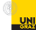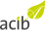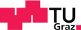## Enantioselectivity: Calculation of E-value

#### Results

Colour
GrayscaleNote Enter two input values to calculate the other parameters. Only values from 1-99 are valid.

Note e.e. = enantiomeric excess [%]; E-value = Enantioselectivity expressed as Enantiomeric Ratio.

Note If e.e. Product and conversion are used to calculate the E-value, the conversion has to be greater than or equal to 30%. If e.e. Substrate and conversion are used to calculate the E-value, the conversion has to be less than or equal to 70%.© Department of Chemistry University Graz, 2013© Austrian Centre for Industrial Biotechnology© Institute for Genomics and Bioinformatics Graz University of Technology, 2013